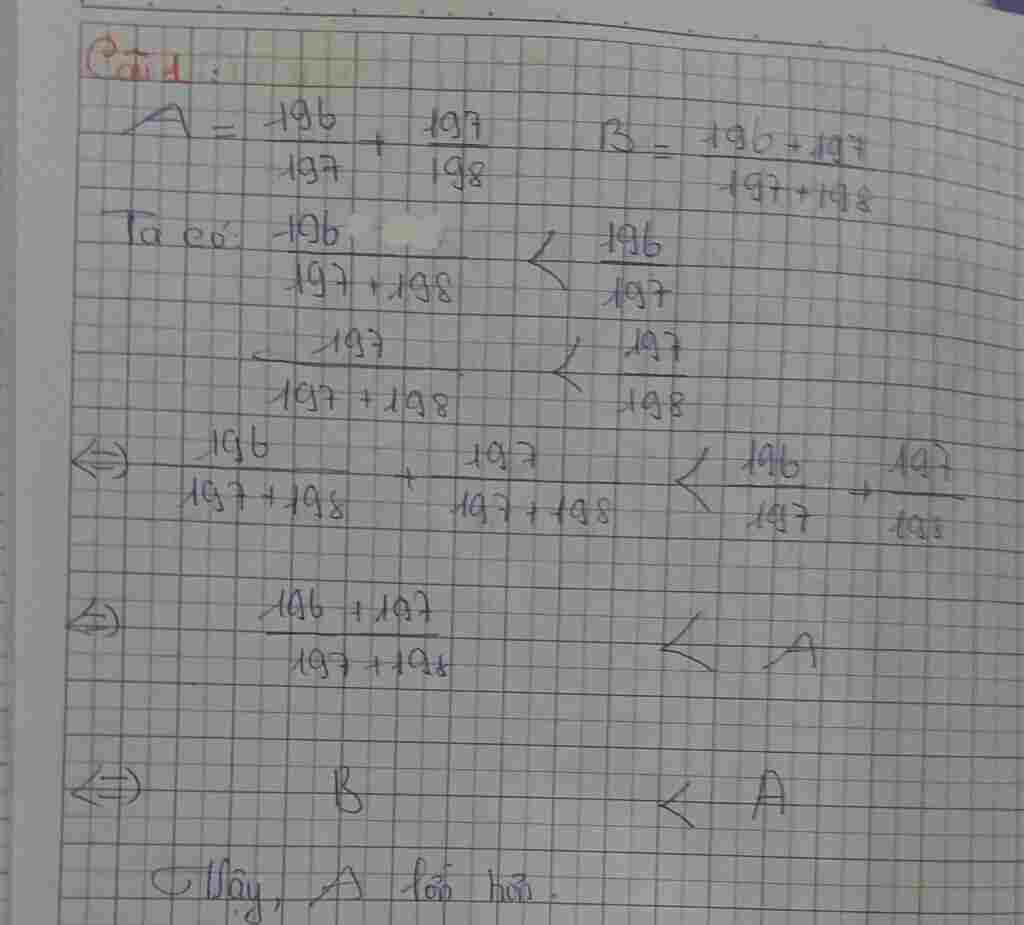## C1:cho A=196/197+197/198 B=197+197/197+198 so nao lon hon C2:1/4+1/5+1/6+…+1/19 chung to B<1 C3:B=1/2^2+1/3^2+1/4^2+1/5^2+1/6^2+1/7^2+1/8^2 chung to

Question

C1:cho A=196/197+197/198 B=197+197/197+198 so nao lon hon
C2:1/4+1/5+1/6+…+1/19 chung to B<1 C3:B=1/2^2+1/3^2+1/4^2+1/5^2+1/6^2+1/7^2+1/8^2 chung to B<1 giup mik voi minh cam on truoc

in progress 0
6 months 2021-05-17T11:00:32+00:00 1 Answers 5 views 0